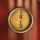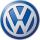# Calculator 2nd power and order of evaluation

### 6^2-(2 * 7 + 4^2) = 6

Spelled result in words is six.

### Calculation steps

1. Exponentiation: 6 ^ 2 = 36
2. Multiple: 2 * 7 = 14
3. Exponentiation: 4 ^ 2 = 16
4. Add: the result of step No. 2 + the result of step No. 3 = 14 + 16 = 30
5. Subtract: the result of step No. 1 - the result of step No. 4 = 36 - 30 = 6
The calculator makes basic and advanced operations with decimals, real numbers and integers. It also shows detailed step-by-step information about calculation procedure. Solve problems with two, three or more decimals in one expression. Add, subtract and multiply decimals step-by-step. This calculator uses addition, subtraction, multiplication or division for calculations on positive or negative decimal numbers, integers, real numbers and whole numbers. This online decimals calculator will help you understand how to add, subtract, multiply or divide decimals.The calculator follows well-known rules for order of operations. The most common mnemonics for remembering this order of operations are:
PEMDAS - Parentheses, Exponents, Multiplication, Division, Addition, Subtraction.
BEDMAS - Brackets, Exponents, Division, Multiplication, Addition, Subtraction
BODMAS - Brackets, Of or Order, Division, Multiplication, Addition, Subtraction.
GEMDAS - Grouping Symbols - brackets (){}, Exponents, Multiplication, Division, Addition, Subtraction.
Be careful, always do multiplication and division before addition and subtraction. Some operators (+ and -) and (* and /) has the same priority and then must evaluate from left to right.

## Decimals in word problems:

• FactorsCan the expression ? be factored into rational factors?
• Parallelogram - sideCalculate the length of the side of a parallelogram whose area is 175 dm2 and height to that side is 24.2 dm long.
• Car factoryThe carmaker now produces 2 cars a day more than last year, so the production of 70312 cars will save just one full working day. How many working days needed to manufacture 70312 cars last year?
• FloorThe floor area of ​​the room is 31 m2 and has a width of 4.3 m. How many centimeters of circumference measured the floor on the map at the scale 1:75?
• ScaleThe swimming pool is long 110 m and 30 m wide. The plan of the city is shown as a rectangle with an area 8.25 cm2. What scale is the city plan?
• Rectangular cuboidThe rectangular cuboid has a surface area 5334 cm2, and its dimensions are in the ratio 2:4:5. Find the volume of this rectangular cuboid.